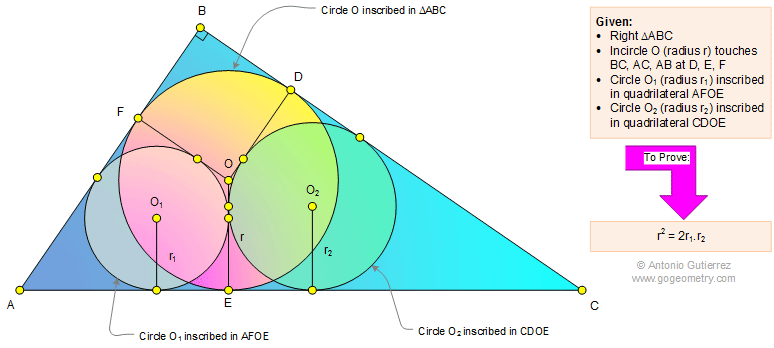Problem 1103: Right Triangle, Incircle, Inscribed Circle, Radius, Geometric Mean, Sangaku, Japanese, Metric Relations. Level: High School, SAT Prep, College Geometry

< PREVIOUS PROBLEM  |  NEXT PROBLEM >

 In a right triangle ABC (see the figure below) incircle O (radius r) is tangent to BC, AC, and AB at D, E, and F, respectively. Circle O1 (radius r1) is inscribed in quadrilateral AFOE and circle O2 (radius r2) is inscribed in quadrilateral CDOE. Prove that r2 = 2r1.r2.See also: Art and typography of problem 1103.
 Home | Search | Geometry | Problems | All Problems | Open Problems | Visual Index | 1101-1110 | Triangle | Right Triangle | Circle | Incircle | Tangent Line | Geometric Mean | Similarity | Sangaku Japanese Geometry | Email | Post a comment or solutionLast updated: Mar 25, 2015, by Antonio Gutierrez